# Traveling Sprinkler Water Application Rate

Use this form to calculate the water application for a traveling big gun sprinkler based on the flow rate, travel speed, and travel lanes.

## Traveling Big Gun Sprinkler Water Application

Flow Rate:
Irrigation Efficiency:
(Minimum efficiency of DuCaR Sprinklers is 80%)
%
Travel Lanes Width:
Travel Speed:
Net Water Application:

### The Equation

This calculator uses this formula to determine the Net Water Application Rate of a traveling big gun sprinkler.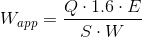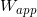= Net Water Application (in)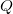= Flow Rate from the sprinkler(gpm)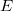= Irrigation Efficiency (as a decimal)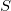= Travel Speed of the Big Gun Sprinkler (ft/min)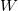= Travel lanes width (ft)

Reference: Washington State University